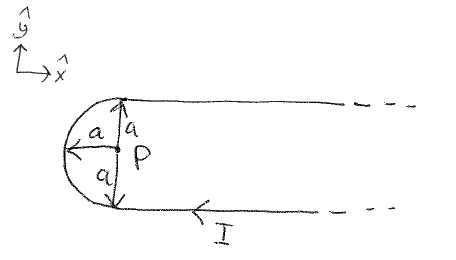# Magnetic Field, Biot-Savart Law & Current-carrying Wire

Sudharshan

## Homework Statement

A current I carried in a conduit as described below. The wire can be assumed to be infinitely long in the positive x-direction. The point P is the radius distance a from the portion of the line that describes a semicircle. Calculate the resulting magnetic field at point P in magnitude and direction.## Homework Equations

Biot-Savart Law:## The Attempt at a Solution

I have calculated the magnetic field caused by the semicircle. I am not quite sure how to calculate the magnetic fields caused by the two infinitely-long wire segments. From my calculations they cancel each other out. Is this correct? Is the magnetic field caused by the semicircle correct?

Page 1:http://i.imgur.com/sPh6NiO.jpg
Page 2:http://i.imgur.com/YOSuQ95.jpg
Page 3:http://i.imgur.com/wL1DCmv.jpg

Any help is appreciated.

Last edited:

## Answers and Replies

Homework Helper
Gold Member
Hello, Sudharshan.

You should be able to decide if the fields of the two straight line segments cancel by using the right hand rule for the direction of magnetic field of a straight section of wire.

Check your signs when setting up the expression for ##\vec{r} - \vec{r}'## in the calculation of the field of L1.

Otherwise, your calculations are very nice and complete!

Sudharshan
Hey TSny,

Does this mean my BL1 is incorrect which leads to the fact that my total magnetic field B is incorrect? Can you specify what mistake you have found? When I calculated BL1 I was unsure about the signs of r and r'.

Last edited:
Homework Helper
Gold Member
The only mistake that I see is in your expression for ##\vec{r}-\vec{r}'## for L1. In particular, what is the sign of the y component of ##\vec{r}##?

Sudharshan
That was the sign I was not sure about. I had written r = ay. Should it not be r = a(-y) because it points in the negative Y direction hence the negative sign?

This should mean that the fields of the two straight line segments do not cancel each other because they point in the same direction with the help of the right hand rule (point towards I with thumb and curl fingers in direction of B).

Sudharshan
So it is only that negative sign that is wrong ? Do you see any other mistakes in the rest of the answer?

Sudharshan
Ok, I will do this change and rewrite my answer. It would be great if you could take another look then and let me know if it is correct. Thanks again for all your help.

Homework Helper
Gold Member
Looks correct. Nice work!

Sudharshan
Thank you.